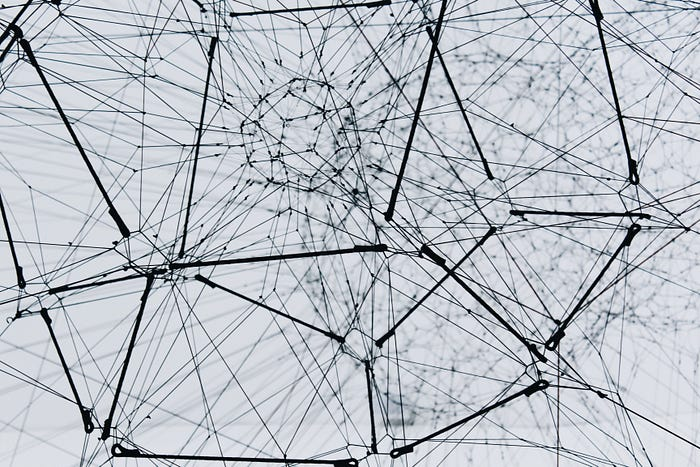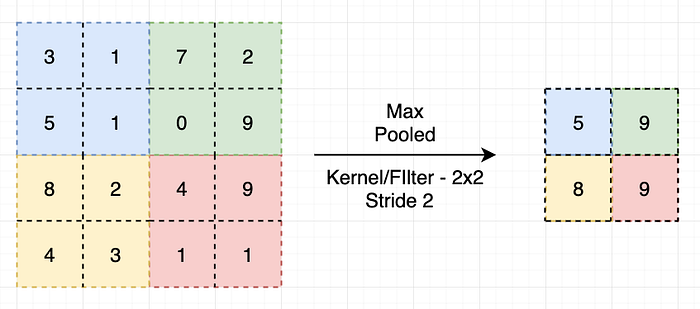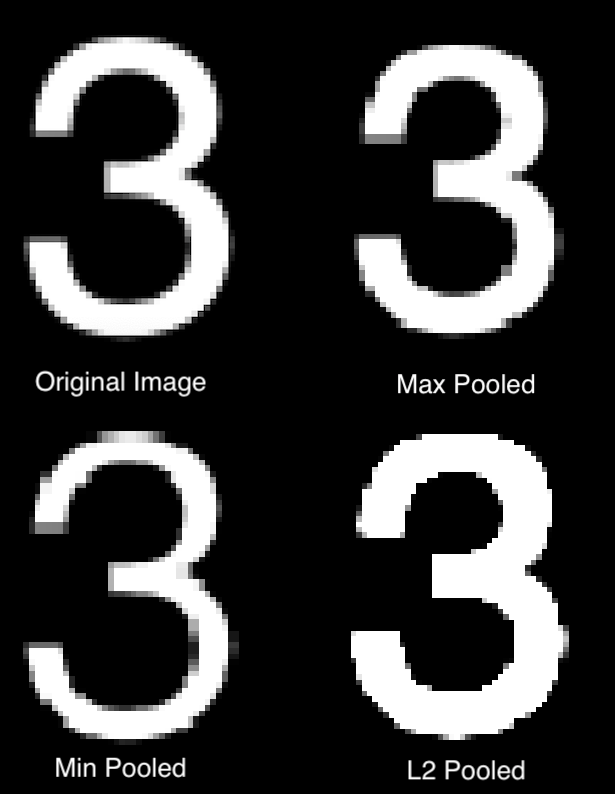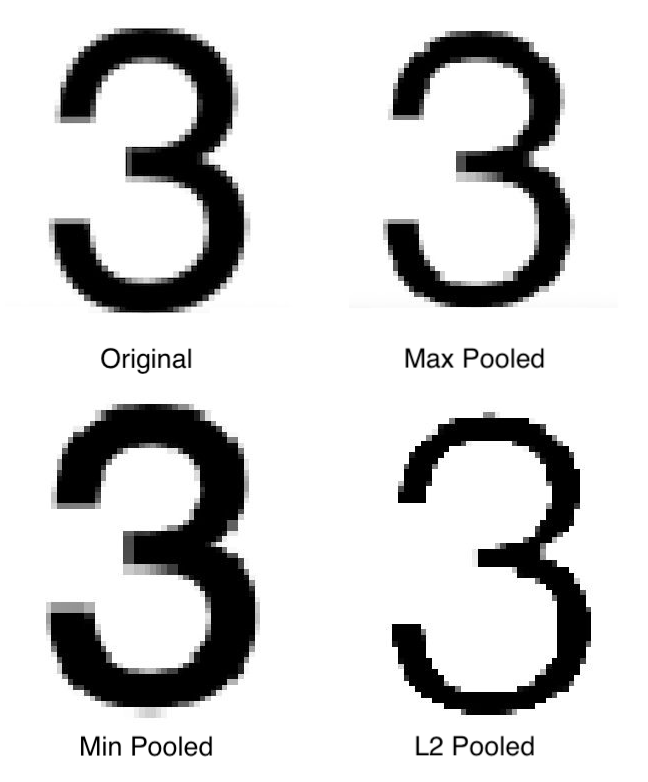# Pooling Layer — Short and Simple

## Here's all the information you should know about Pooling Layer in CNNThis story will answer the following points.

1. What is pooling layer?
2. Formula for output volume of data when pooling is applied.
4. Is the pooling layer trained during the backpropagation of loss?
5. Types of pooling layer with examples.
6. Can we remove the pooling layer?

The pooling layer is commonly applied after a convolution layer to reduce the spatial size of the input. It is applied independently to each depth slice of the input volume. Volume depth is always preserved in pooling operation.

Kernel/filter is the size of a matrix that is applied over the complete input data.Max Pooling layer applied a single slice of an input volume.

### Formula

Assume we have an input volume of width W¹, height H¹, and depth D¹. The pooling layer requires 2 hyperparameters, kernel/filter size F and stride S.

On applying the pooling layer over the input volume, output dimensions of output volume will be

W² = (W¹-F)/S + 1
H² = (H¹-F)/S + 1
D² = D¹

For the pooling layer, it is not common to pad the input using zero-padding.

### Advantages of the pooling layer

1. Reduces the number of training parameters and computation cost, thus control overfitting.
2. Make model invariant to certain distortion.

### Is the pooling layer trained during backpropagation?

The pooling layer is not trained during the backpropagation of gradients because the output volume of data depends on the values of the input volume of data.

### Types of Pooling Layer

1. Max Pooling: In this type of pooling, the maximum value of each kernel in each depth slice is captured and passed on to the next layer.
2. Min Pooling: In this type, the minimum value of each kernel in each depth slice is captured and passed on to the next layer.
3. L2 Pooling: In this type, the L2 or the Frobenius norm is applied to each kernel.
4. Average Pooling: In this type, the average value of the kernel is calculated.

I've applied three kernels i.e. Max, Min, and L2 on two images. The following are the outputs.Collage 1Collage 2

### Comparison

1. Every image is composed of pixels whose values range from 0–255. 0 is black and 255 is white.
2. Both original images in the above collages are grayscaled images. The original image of Collage 1 is white text on a black background, while the original image of Collage 2 is black text on a white background.
3. Max pooling takes the maximum value of a section, therefore, the “max pooled image” of collage 1 is similar to the original image because the white pixel values (text area) are given more importance than black pixel values (background).
While “max pooled image” of collage 2 is shrunk in size because white pixel values (background area) are given importance than white pixel values (text area).
4. Min pooling takes the minimum value of a section, therefore the “min pooled image” of collage 1 is shrunk while the “min pooled image” of collage 2 looks similar to the original image of collage 2.
5. “L2 pooled” smoothened the curves of the text area in both images and retained the properties of “max pooled” of respective original images.

### Ever thought of getting rid of the pooling layer?

Striving for Simplicity: The All Convolution Net found that the max-pooling layer can be replaced with a convolution layer with increased stride without compromising the accuracy.

Congratulations on making it to the end. Thank you for reading!